Applications of Systems of Equations with Three Variables

Applications of Systems of Equations
with Three Variables

It is often necessary to use more than one variable to model a situation in a field such as business, science, psychology, finance, engineering, or other disciplines.  Applying the skills of solving a system of equations can help solve these types of problems.

Example

Marcy inherited \$25,000 and invested part of it in a money market account, part in municipal bonds, and part in a mutual fund. After one year, she received a total of \$1,620 in simple interest from the three investments. The money market paid 6% annually, the bonds paid 7% annually, and the mutual fund paid 8% annually. There was \$6,000 more invested in the bonds than the mutual funds. Find the amount Marcy invested in each category.

Understand

Let :

x = The amount of money invested in the money market account.
y = The amount of money invested in municipal bonds.
z = The amount of money invested in a mutual fund.

The total investment is represented as:

x + y + z = \$25,000

The interest earned can be represented as:

0.06x + 0.07y + 0.08z = \$1,620

The difference in the amounts invested in municipal bonds and mutual funds can be represented as:

y - z = \$6,000

Process

The system of three equations can now be solved either by substitution, elimination, or with matrices.  Any process appropriately applied will supply a correct answer.  For this example, let's solve the problem by using matrices.

Solving with Matrices

The process of using matrices is essentially a shortcut for the process of elimination. Each row of the matrix represents an equation, and each column represents coefficients of one of the variables.

Step 1:  Create a three-row by four-column matrix using coefficients and the constant of each equation.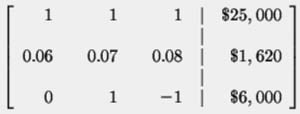The vertical dashed line in the matrix stands for the equal signs between both sides of each equation. The first column contains the coefficients of x, the second column contains the coefficients of y, the third column contains the coefficients of z, and the last column contains the constants.

We want to convert the original matrix to the following matrix: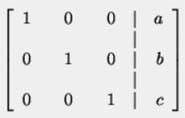because you can
then read the matrix as x = a, y = b, and z = c.

Step 2:  We work with column 1 first. The number 1 is already in Cell 11 (That's not "eleven," it's "one-one," as in "Row 1, Column 1").

Add -0.06 times Row 1 to Row 2 to form a new Row 2.

-0.06 [Row 1] + [Row 2] = [New Row 2]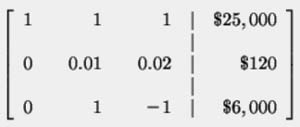Step 3:  We will now work with column 1. We want 1 in Cell 22, and we achieve this by multiplying Row 2 by 100.

100[Row 2] = [New Row 2]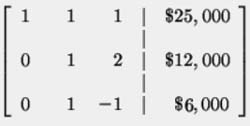Step 4:  Let's now manipulate the matrix so that there are zeros in Cell 12 and Cell 32. We do this by adding the opposite of Row 2 to Row 1 and the opposite of Row 2 to Row 3. That gives us a new Row 1 and a new Row 3.

- [Row 2] + [Row 1] = [New Row 1]
- [Row 2] + [Row 3] = [New Row 3]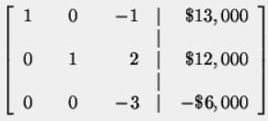Step 5:  Let's now manipulate the matrix so that there is a 1 in Cell 33. We do this by multiplying Row 3 by -1/3.

-1/3 [Row 3] = [New Row 3]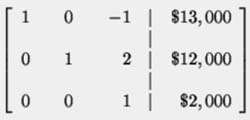Step 6:  Let's now manipulate the matrix so that there are zeros in Cell 13 and Cell 23. We do this by adding Row 3 to Row 1 for a new Row 1 and adding -2 times Row 3 to Row 2 for a new Row 2.

1[Row 3] + [Row 1] = [New Row 1]
-2[Row 3] + [Row 2] = [New Row 2]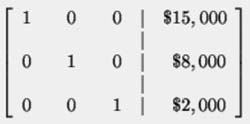Solution